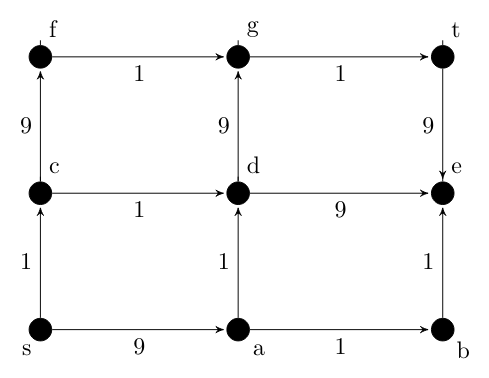# GATE | GATE CS 2021 | Set 2 | Question 65

• Difficulty Level : Basic
• Last Updated : 23 May, 2021

In a directed acyclic graph with a source vertex s, the quality-score of a directed path is defined to be the product of the weights of the edges on the path. Further, for a vertex v other than s, the quality-score of v is defined to be the maximum among the quality-scores of all the paths from s to v. The quality-score of s is assumed to be 1.The sum of the quality-scores of all vertices on the graph shown above is _______ .
(A) 929
(B) 81
(C) 729
(D) 1023

Explanation: Let Q(V) denote the quality-score of vertex V.

```Q(S) = 1 (Given)
Q(C) = 1 (S → C)
Q(F) = 1 * 9 (S  → C  → F)
Q(A) = 9 (S  → A)
Q(D) = 9*1 (S  → A  → D)
Q(G) = 9 * 1 * 9 (S  → A  → D  → G)
Q(B) = 9 * 1 (S  → A  → B)
Q(E) = 9 * 1 * 9 (S  → A  → D  → E)
Q(T) = 9*1*9*9 (S  → A  → D  → E → T) ```

Sum of quality-score of all vertices,

```= 1 + 1 + 9 + 9 + 9 + 81 + 9 + 81 + 729
= 929 ```
My Personal Notes arrow_drop_up
Recommended Articles
Page :